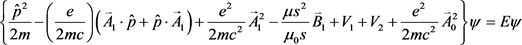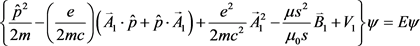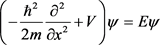# A-B效应中不可积相位因子的导出Derivation of the Non-Integrable Phase Factor of A-B Effect

DOI: 10.12677/APP.2020.106039, PDF, HTML, XML, 下载: 49  浏览: 125

Abstract: Most of studies about the derivation of the non-integrable phase factor of A-B effect are in the way of path integral at present, but the physical process is not easy to understand. Base on the Schrö-dinger equation, the non-integrable phase factor is obtained in a simple way. And from the theoretical analysis the result that the essence of the non-integrable phase factor is the wave-particle duality of microscopic objects is obtained.

1. 引言

2. 不可积相因子的导出

$\stackrel{^}{H}=\frac{{\stackrel{^}{p}}^{2}}{2m}-\left(\frac{e}{2mc}\right)\left(\stackrel{\to }{A}\cdot \stackrel{^}{p}+\stackrel{^}{p}\cdot \stackrel{\to }{A}\right)+\frac{{e}^{2}}{2m{c}^{2}}{\stackrel{\to }{A}}^{2}-\frac{\mu \stackrel{^}{s}}{{\mu }_{0}s}\cdot \stackrel{\to }{B}$ (1)

${\stackrel{^}{H}}_{1}=\frac{{\stackrel{^}{p}}^{2}}{2m}-\left(\frac{e}{2mc}\right)\left(\stackrel{\to }{A}\cdot \stackrel{^}{p}+\stackrel{^}{p}\cdot \stackrel{\to }{A}\right)+\frac{{e}^{2}}{2m{c}^{2}}{\stackrel{\to }{A}}^{2}-\frac{\mu \stackrel{^}{s}}{{\mu }_{0}s}\cdot \stackrel{\to }{B}+{V}_{1}$ (2)

${\stackrel{^}{H}}_{2}=\frac{{\stackrel{^}{p}}^{2}}{2m}-\left(\frac{e}{2mc}\right)\left(\stackrel{\to }{A}\cdot \stackrel{^}{p}+\stackrel{^}{p}\cdot \stackrel{\to }{A}\right)+\frac{{e}^{2}}{2m{c}^{2}}{\stackrel{\to }{A}}^{2}-\frac{\mu \stackrel{^}{s}}{{\mu }_{0}s}\cdot \stackrel{\to }{B}+{V}_{2}$ (3)

$\stackrel{^}{H}={\stackrel{^}{H}}_{1}+{\stackrel{^}{H}}_{2}$ (4)

$\stackrel{⇀}{H}=\frac{{\stackrel{^}{p}}^{2}}{m}-\left(\frac{e}{mc}\right)\left[\left({\stackrel{\to }{A}}_{0}+{\stackrel{\to }{A}}_{1}\right)\cdot \stackrel{^}{p}+\stackrel{^}{p}\cdot \left({\stackrel{\to }{A}}_{0}+{\stackrel{\to }{A}}_{1}\right)\right]+\frac{e{}^{2}}{m{c}^{2}}{\left({\stackrel{\to }{A}}_{0}+{\stackrel{\to }{A}}_{1}\right)}^{2}-\frac{2\mu \stackrel{^}{s}}{{\mu }_{0}s}\cdot \left({\stackrel{⇀}{B}}_{0}+{\stackrel{\to }{B}}_{1}\right)+{V}_{1}+{V}_{2}$(6)(7)$\left\{\frac{{\stackrel{^}{p}}^{2}}{2m}-\left(\frac{e}{2mc}\right)\left({\stackrel{⇀}{A}}_{1}\cdot \stackrel{^}{p}+\stackrel{^}{p}\cdot {\stackrel{\to }{A}}_{1}\right)+\frac{{e}^{2}}{2m{c}^{2}}{\stackrel{\to }{A}}_{1}-\frac{\mu {s}^{2}}{{\mu }_{0}s}{\stackrel{⇀}{B}}_{1}+{V}_{1}\right\}{\psi }_{0}=E{\psi }_{0}$ (8)

3. 理论分析

4. 结论

  Tonomura, A., Osakabe, N., et al. (1986) Evidence for Aharonov-Bohm Effect with Magnetic Field Completely Shielded from Electron Wave. Physical Review Letters, 56, 792. https://doi.org/10.1103/PhysRevLett.56.792  王瑞峰. A-B效应的动力学机制及其实验验证方案[J]. 物理学报, 2005, 54(10): 4532-4537.  朗道. 非相对论量子力学[M]. 第六版. 北京: 高等教育出版社, 2008: 413.  Wu, T.T. and Yang, C.N. (1975) Concept of Nonintegrable Phase Factors and Global Formulation of Gauge Fields. Physical Review D, 12, 3845. https://doi.org/10.1103/PhysRevD.12.3845  Berry, M.V. (1984) Quantal Phase Factors Accompanying Adiabatic Changes. Proceedings of the Royal Society of London, A392, 45. https://doi.org/10.1098/rspa.1984.0023  Berry, M.V. (1990) Anticipations of the Geometric Phase. Physics Today, 43, 34. https://doi.org/10.1063/1.881219  Wang, Z.-C., Su, G., Li, L. and Gao, J. (2004) The Effect of the Geometric Phase on Spin-Polarized Electron Tunnelling. Journal of Physics: Condensed Matter, 16, 6713-6726. https://doi.org/10.1088/0953-8984/16/37/007